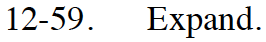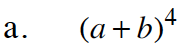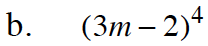Home > CCA2 > Chapter 12 > Lesson 12.1.3 > Problem12-59

12-59.
1. Expand. Homework Help ✎

1. (a + b)4

2. (3m − 2)4For help with this problem, refer to problem 12-22 in Lesson 12.1.1.

$a^4+4a^3b+6a^2b^2+4ab^3+b^4$Use the results of part (a). Let a = 3m and b = −2. Simplify.

$81m^4 -216m^3 +216m^2 -96m+16$